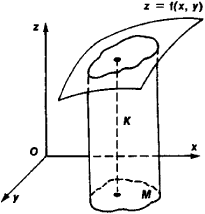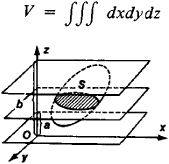# volume

(redirected from hypervolemia)
Also found in: Dictionary, Thesaurus, Medical, Legal, Financial, Wikipedia.

See also: Formulas for the Volumes of Some Common Solids (table)Formulas for the Volumes of Some Common Solids
Solid Volume1
cube l3
right rectangular parallelepiped lwh
prism Bh
right circular cylinder πr2h
pyramid 1-3Bh

## volume,

measure of solid content or capacity, usually expressed in units that are the cubes of linear units, such as cubic inches and cubic centimeters, or in units of dry and liquid measure, such as bushels, gallons, and liters. Volumes of complicated geometric forms are often calculated using integral calculuscalculus,
branch of mathematics that studies continuously changing quantities. The calculus is characterized by the use of infinite processes, involving passage to a limit—the notion of tending toward, or approaching, an ultimate value.
. See the table entitled Formulas for the Volumes of Some Common SolidsFormulas for the Volumes of Some Common Solids
Solid Volume1
cube l3
right rectangular parallelepiped lwh
prism Bh
right circular cylinder πr2h
pyramid 1-3Bh
.

## Volume

one of the fundamental quantities associated with geometric solids. In the simplest cases volume is measured by the number of unit cubes that can fit in the solid, that is, the number of cubes with an edge of unit length.

The problem of calculating the volumes of very simple solids stems from practical needs and was a stimulus to the development of geometry. The mathematics of the ancient East—Babylonia and Egypt—had a number of mostly empirical rules for calculating the volume of such frequently encountered solids as prismatic beams, whole and truncated pyramids, and cylinders. The volume formulas included inaccurate ones. The percentage error resulting from the use of inaccurate formulas was slight only within the limits of the linear dimensions commonly encountered in solids.

The Greek mathematicians of the last centuries before the Common Era freed the theory of volume calculation from approximate empirical rules. Euclid’s Elements and the works of Archimedes contain only exact rules for calculating the volumes of polyhedrons and certain round solids, namely, the cylinder, cone, sphere, and parts thereof. In their theory of volumes of polyhedrons, Greek mathematicians had to overcome the considerable difficulties that make this branch of geometry substantially different from the related branch dealing with the areas of polygons. Not until the early 20th century was the reason for this difference found. Using rectilinear cuts it is possible to dissect a polygon into parts that can be rearranged into a square. Dehn showed in 1901 that the analogous transformation of an arbitrary polyhedron into a cube by means of plane cuts is in general impossible. That is why, in establishing the formula for the volume of a tetrahedron, the simplest of all polyhedrons, Euclid had to resort to an infinite process of successive approximations—the method of exhaustion.

An infinite process also underlies the modern treatment of volume measurement. The modern approach considers all possible polyhedrons inscribed in the solid K and all possible polyhedrons circumscribed about K. The calculation of the volume of a polyhedron reduces to the calculation of the volume of the polyhedron’s constituent tetrahedrons. Let {Vi} be the set of volumes of the polyhedrons inscribed in K and {Vd} be the set of volumes of the polyhedrons circumscribed about K. The set {Vi} is bounded from above by the volume of any circumscribed polyhedron, and the set {Vd} is bounded from below by, for example, the number 0. The least upper bound of {Vi} is called the inner volume V̱ of K, and the greatest lower bound of {Vd} is called the outer volume V̱ of K. If \$V coincides with V, then the number V = V = V is called the volume of K, and K is said to be Jordan measurable. A necessary and sufficient condition for a solid to be measurable is that for any positive number ∊ there exist a polyhedron circumscribed about the solid and a polyhedron inscribed in the solid such that the difference VdVi of their volumes is less than ∊.

Analytically, volume can be expressed by means of multiple integrals. Let the solid K (Figure 1) be bounded by a cylindrical surface with generators parallel to the z-axis, by the Jordan-measurable region M of the plane xOy, and by the surfaceFigure 1

z = f(x, y), which is intersected by any line parallel to a generator of the cylinder at exactly one point. The volume of such a solid can be calculated by means of the double integral

V = ∫∫Mf (x, y) dxdy

The volume of a solid bounded by a closed surface that meets a line parallel to the z-axis at no more than two points can be calculated as the difference of the volumes of two solids of the kind just described. The volume of a solid can also be expressed by the triple integralFigure 2

where the integration extends over the part of space occupied by the solid. Sometimes it is convenient to calculate the volume of a solid in terms of cross sections. Let a solid (Figure 2) lying between the planes z = a and z = b, b > a, be cut by planes perpendicular to the z-axis. If all cross sections of the solid are measurable and the cross-sectional area S is a continuous function of z, then the volume of the solid can be expressed by the simple integralHistorically, the operation of integration was actually used (in various geometric forms) to calculate the volumes of simple solids—the pyramid, the sphere, and some solids of revolution—long before the development of the integral calculus. Thus the way was prepared for the development of the integral calculus in the 17th and 18th centuries. In particular, Cavalieri’s principle, which is still important as a teaching device, contained formula (1) in embryonic form. Simpson’s rule, which is appropriate when the function S(z) in (1) is a polynomial of at most third degree, is also useful in elementary instruction.

### REFERENCES

Kudriavtsev, L. D. Matematicheskii analiz, vols. 1–2. Moscow, 1970.
Lebesgue, H. Ob izmerenii velichin, 2nd ed. Moscow, 1960. (Translated from French.)

## volume

[′väl·yəm]
(acoustics)
The intensity of a sound.
(computer science)
A single unit of external storage, all of which can be read or written by a single access mechanism or input/output device.
(engineering acoustics)
The magnitude of a complex audio-frequency current as measured in volume units on a standard volume indicator.
(mathematics)
A measure of the size of a body or definite region in three-dimensional space; it is equal to the least upper bound of the sum of the volumes of nonoverlapping cubes that can be fitted inside the body or region, where the volume of a cube is the cube of the length of one of its sides. Abbreviated vol.

## volume

1. the magnitude of the three-dimensional space enclosed within or occupied by an object, geometric solid, etc.
2. fullness or intensity of tone or sound
3. History a roll or scroll of parchment, papyrus, etc.

## volume

A physical storage unit, such as a hard disk, floppy disk, disk cartridge, CD-ROM disc or reel of tape. See volume label and logical volume.
References in periodicals archive ?
2005) Sodium-facilitated hypervolemia, endurance performance, and thermoregulation.
As an example, hypervolemia is not as pronounced in supine cycling (18) or swimming (15) compared with upright exercise and training mode-specific differences also exist between runners and swimmers in regard to alterations in cardiac dimension (19).
Factors that may be related to death in this group during the infection are mainly due to the physiological changes of pregnancy, such as (I) reduced pulmonary functional capacity caused by compression of visceral structures by the pregnant uterus; (II) hypervolemia, which would decrease the capacity of hemodynamic adaptation in case of sepsis; and (III) immunological impairment of cell-mediated immunity attributed to immune tolerance to paternal antigens of the fetus, which would limit the immunity against the flu virus.
Fluid retention associated with CHF induces hypervolemia.
The vasospasm was treated with induced hypertension and hypervolemia, using norepinephrine and dobutamine drips to maintain a systolic BP of 160-180 mm Hg as well as packed red blood cells and isotonic fluids to keep her central venous pressure (CVP) >8 mm Hg.
Disorders related to fluid and electrolyte imbalance may include hypervolemia, hyperkalemia, nausea and vomiting, hyperparathyroidism, bone disease and hypertension (Mitch, 2005; Snively, 2004).
Although the hypervolemia and tachycardia may exacerbate mitral valve obstruction, echocardiographic measurement of mitral stenosis severity including 2D valve area, Doppler gradients and pressure half time valve area, remain valid in pregnancy allowing optimization of patient monitoring and therapy (8).
Of note, poor metabolic control per se increases cardiac output by ~10% in adults with type 1 diabetes (30), and it is conceivable that the increase in fetal BNP secretion in infants of mothers with diabetes reflects increased pre- or afterload of the fetal heart, perhaps attributable to fetal hypervolemia.
There were 35 patients who qualified for surgical treatment consisting of early surgery, prophylactic hypervolemia, and aggressive treatment of vasospasm, and 19 patients who received palliative care only.
If the indwelling catheter is patent and the patient demonstrates signs and symptoms of hypervolemia (elevated CVP and blood pressure, edema, distended neck veins), up to 200 mg of furosemide should be administered intravenously.

Site: Follow: Share:
Open / Close